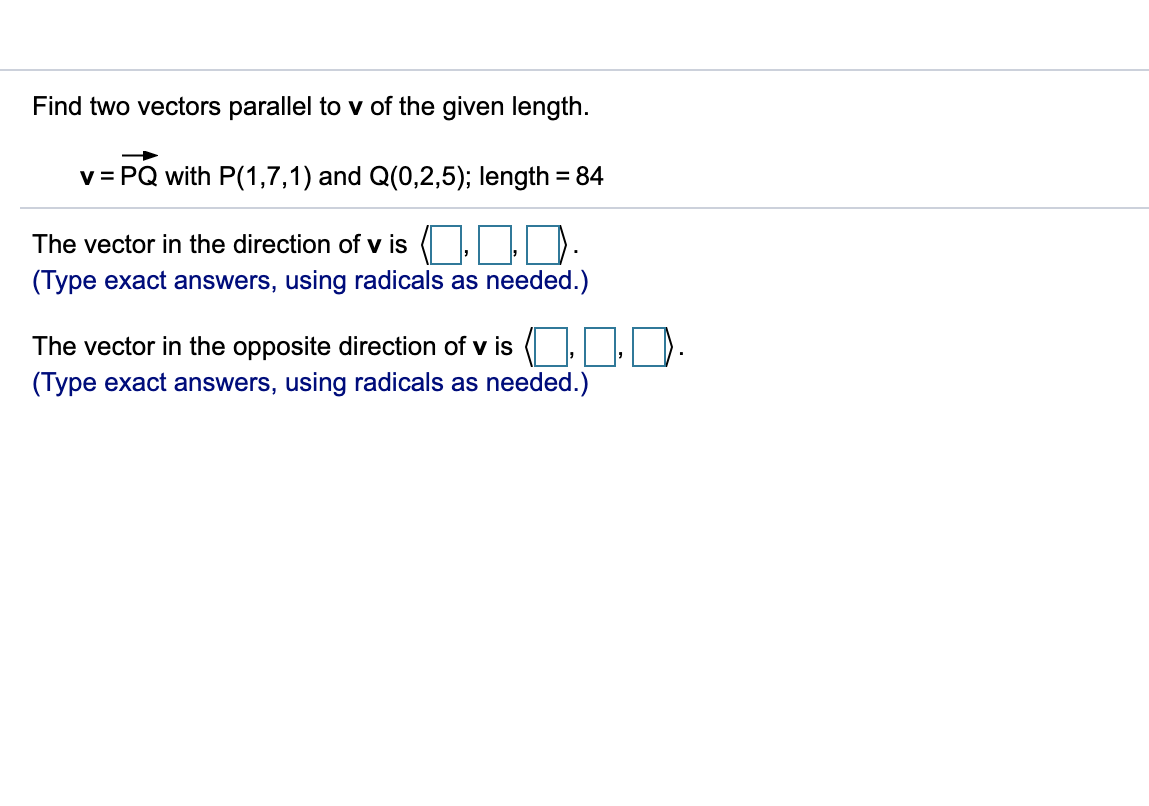# Find two vectors parallel to v of the given length.v PQ with P(1,7,1) and Q(0,2,5); length 84The vector in the direction of v is(Type exact answers, using radicals as needed.)The vector in the opposite direction of v is(Type exact answers, using radicals as needed.)

Question
1 viewshelp_outlineImage TranscriptioncloseFind two vectors parallel to v of the given length. v PQ with P(1,7,1) and Q(0,2,5); length 84 The vector in the direction of v is (Type exact answers, using radicals as needed.) The vector in the opposite direction of v is (Type exact answers, using radicals as needed.) fullscreen
check_circle

Step 1

firstly, we find vector v , that is vector PQ, which is equal to (position vector of Q)-(position vector of P)

Step 2

We know that any vector parallel to a given vector is m times that vector , where m is any scalar.

Let vector a is parallel to vector v

Step 3

Since length of vector a is 84 , th...

### Want to see the full answer?

See Solution

#### Want to see this answer and more?

Solutions are written by subject experts who are available 24/7. Questions are typically answered within 1 hour.*

See Solution
*Response times may vary by subject and question.
Tagged in

### Calculus